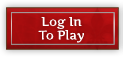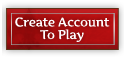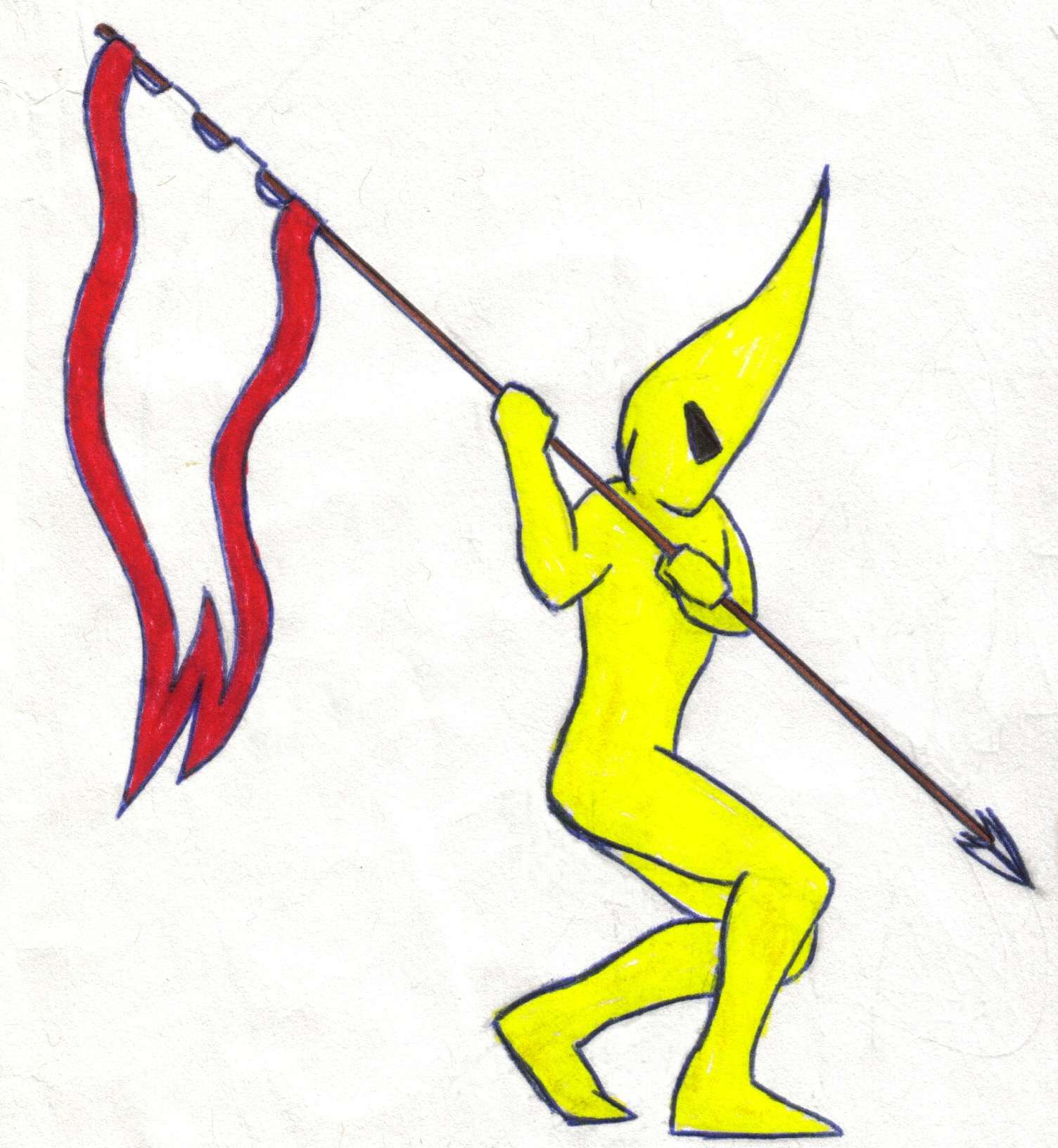Forum Home > News & Announcements > AnnouncementsNew PostsFAQSearchRegisterLogin

#Mild Release: Patch sizes, Animal parts

 Post ReplyPage  123>
AuthorTopic SearchTopic Options
abstractdreamPostmaster GeneralJoined: 02 Oct 2011
Location: Oarnamly
Status: Offline
Points: 1843Topic: Mild Release: Patch sizes, Animal parts
Posted: 19 Aug 2012 at 21:40
I want to thank you ALL for your dedication and hard work. The list is quite useful and I go back to it almost every day.

I bookmark the IGM with the codes for quick reference.

Thanks again.
Bonfyr VerbooElf DragonGreenhornJoined: 18 Apr 2012
Status: Offline
Points: 58Posted: 19 Aug 2012 at 21:25
Thanks for that :)WonderingNew PosterJoined: 18 Feb 2012
Status: Offline
Points: 6Posted: 19 Aug 2012 at 20:36
The game icons are in sprite format, which while great for game speed, makes it difficult for 3rd party tools and fansites.

Can individual icons be created for player use, perhaps added to the updated fansite package?tallicaForum WarriorJoined: 27 Jun 2011
Location: Seattle, WA
Status: Offline
Points: 378Posted: 19 Aug 2012 at 17:03dunnoob wrote:to Mogul, Quackers, Salarius, and Tallica; I've copied the list in an IGM.  Is it only me, or does everybody send lots of IGMs to themselves for archiving?

That's what I did with my set, well, just the crafting items so that I have a quick reference at hand.dunnoobPostmasterJoined: 10 Dec 2011
Location: Elijal
Status: Offline
Points: 800Posted: 19 Aug 2012 at 03:07to Mogul, Quackers, Salarius, and Tallica; I've copied the list in an IGM.  Is it only me, or does everybody send lots of IGMs to themselves for archiving?

Edited by dunnoob - 19 Aug 2012 at 03:08tallicaForum WarriorJoined: 27 Jun 2011
Location: Seattle, WA
Status: Offline
Points: 378Posted: 18 Aug 2012 at 22:29
mine has a couple of weapons that were missing from Quackers, so the 2nd one!

Edit: or are you asking for which ones were missed? That question I don't know anymore, spend so long going through them all!

Edited by tallica - 18 Aug 2012 at 22:31SalarariusPostmasterJoined: 26 Sep 2011
Location: USA
Status: Offline
Points: 519Posted: 18 Aug 2012 at 22:18
Which ones? :)

Ah, found them.

Thank You

Edited by Salararius - 19 Aug 2012 at 17:55tallicaForum WarriorJoined: 27 Jun 2011
Location: Seattle, WA
Status: Offline
Points: 378Posted: 18 Aug 2012 at 21:13
Quackers missed a couple of crafted weapons (and maybe armors?) which I've included in this list: (you can copy this to wherever you'd like)

Basic Resources
[@c=183][@c=17][@c=19][@c=21][@c=23][@c=25][@c=295][@c=296]

Advanced Resources
[@c=1][@c=2][@c=12][@c=7][@c=5][@c=3][@c=4][@c=6][@c=8][@c=9][@c=10][@c=11][@c=420]

Minerals
[@c=417][@c=193][@c=194][@c=195][@c=196][@c=197][@c=198][@c=199][@c=200][@c=201][@c=202][@c=203][@c=204][@c=205][@c=206][@c=207][@c=208][@c=209][@c=210][@c=211][@c=212]

Herbs
[@c=416][@c=253][@c=224][@c=225][@c=226][@c=227][@c=228][@c=229][@c=230][@c=231][@c=232][@c=233][@c=234][@c=235][@c=236][@c=237][@c=238][@c=239][@c=240][@c=241][@c=242][@c=243][@c=244][@c=245][@c=246][@c=247][@c=248][@c=249][@c=250][@c=251]
[@c=252]

Animal Parts
[@c=186][@c=298][@c=299][@c=300][@c=301][@c=302][@c=303][@c=304][@c=305][@c=306][@c=307][@c=308][@c=309][@c=310][@c=311][@c=312][@c=313][@c=314][@c=315][@c=316][@c=317][@c=318][@c=319][@c=320][@c=321][@c=322][@c=323][@c=324][@c=325][@c=326][@c=327][@c=328][@c=329][@c=330][@c=331][@c=332][@c=333][@c=334][@c=335][@c=336][@c=337][@c=338][@c=339][@c=340][@c=341][@c=342][@c=343][@c=344][@c=345][@c=346][@c=347][@c=348][@c=349][@c=350][@c=351][@c=352][@c=353][@c=354][@c=355][@c=356][@c=357][@c=358][@c=359][@c=360][@c=361][@c=362][@c=363][@c=364][@c=365][@c=366][@c=367][@c=368][@c=369][@c=370][@c=371][@c=372][@c=373][@c=374][@c=375][@c=376][@c=377][@c=378][@c=379][@c=380][@c=381][@c=382][@c=383][@c=384][@c=385][@c=386][@c=387][@c=388][@c=389][@c=390][@c=391][@c=392][@c=393][@c=394][@c=395][@c=396][@c=397][@c=398][@c=399][@c=400][@c=401][@c=402][@c=403][@c=404][@c=405][@c=406][@c=407][@c=408][@c=409][@c=410][@c=411][@c=412][@c=413][@c=414][@c=415]

[@c=290][@c=291][@c=292][@c=293]

[@c=255][@c=256][@c=257][@c=258]

Swords
[@c=40][@c=41][@c=42][@c=43][@c=44][@c=45][@c=46][@c=47][@c=48][@c=50][@c=51][@c=52][@c=53][@c=54][@c=55][@c=56][@c=57][@c=58][@c=59][@c=60][@c=61][@c=62][@c=63][@c=64][@c=65][@c=49]

Bows
[@c=67][@c=68][@c=69][@c=70][@c=71][@c=72][@c=73][@c=74][@c=75][@c=76][@c=77][@c=78][@c=79][@c=80][@c=81][@c=82][@c=83][@c=84][@c=85][@c=86][@c=87][@c=88][@c=89]

Spears
[@c=91][@c=92][@c=93][@c=94][@c=95][@c=96][@c=97][@c=98][@c=99][@c=100][@c=101][@c=102][@c=103][@c=104][@c=105][@c=106][@c=107][@c=108][@c=109][@c=110][@c=111][@c=112][@c=113][@c=114][@c=115][@c=116]

Horses
[@c=33][@c=34][@c=35][@c=36][@c=37]

[@c=422][@c=423][@c=424][@c=425]

Leather Armors
[@c=120][@c=121][@c=122][@c=123][@c=124][@c=125][@c=126][@c=127][@c=128][@c=129][@c=130][@c=131][@c=132][@c=133][@c=134][@c=135][@c=136][@c=137][@c=138][@c=139]

Chain Armors
[@c=141][@c=142][@c=143][@c=144][@c=145][@c=146][@c=147][@c=148][@c=149][@c=150][@c=151][@c=152][@c=153][@c=154][@c=155][@c=156][@c=157][@c=158][@c=159]

Plate Armors
[@c=161][@c=162][@c=163][@c=164][@c=165][@c=166][@c=167][@c=168][@c=169][@c=170][@c=171][@c=172][@c=173][@c=174][@c=175][@c=176][@c=177][@c=178][@c=179]

Edited by tallica - 18 Aug 2012 at 21:15lokifeysonForum WarriorJoined: 29 Jul 2010
Status: Offline
Points: 211Posted: 03 Aug 2012 at 06:50
dude yes, just made me like the update more :)

i was saying we needed exactly this :)QuackersForum WarriorJoined: 19 Nov 2011
Location: Jeff City
Status: Offline
Points: 435Posted: 03 Aug 2012 at 02:16Salararius wrote:Thanks so much, here is what I was looking for but what you posted had what I didn't know in it.Thanks!

Yea I wanted to give you the code I typed but then I noticed all the blank code that did nothing. Didn't want you to have to shift through and figure all that stuff out as well. :P

Your profile looks great, really nice job with the labeling. Oh and here is your profile if people want to see: http://elgea.illyriad.co.uk/#/Player/Profile/66699
Make it your ambition to lead a quiet life, to mind your own business and to work with your hands, so that your daily life may win the respect of outsiders and so you will not be dependent on anybody.Post ReplyPage  123>Tweet

 Forum Jump -- Select Forum --  News  Announcements   - Contests!  Rules  Help  General Questions  Strategies, Tips & Tricks  Technical Support  Elgea   - Alliance Recruitment   - Politics & Diplomacy   - Trade Chat  Broken Lands   - Alliance Recruitment   - Politics & Diplomacy   - Trade Chat  Events   - Tournament I - Hold the Flags!   - Tournament II - Bloodthirst For Knowledge   - Tournament III - Harness the Elementals   - Tournament IV - The Undead Horde   - Tournament V - 2nd Anniversary   - Tournament VI - Dark Harvest   - Tournament VII - Champions Return   - Tournament VIII - The Ruby Jubilee   - Tournament IX - The Tinkers' Tournament   - Tournament X - The Chill of Winter  Lore   - From the Herald  The Caravanserai  The Travellers' Tale   - Fiction   - Histories   - Art  Suggestions & Game Enhancements   - Implemented  Fansites  Technology & data  UIv2  General Discussion   - Side Quests! Forum PermissionsYou cannot post new topics in this forumYou cannot reply to topics in this forumYou cannot delete your posts in this forumYou cannot edit your posts in this forumYou cannot create polls in this forumYou cannot vote in polls in this forum

Forum Software by Web Wiz Forums® version 11.10
Copyright ©2001-2017 Web Wiz Ltd.